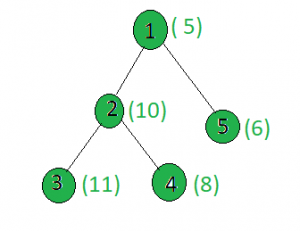# Count the nodes in the given tree whose weight is a power of two

Given a tree, and the weights of all the nodes, the task is to count the number of nodes whose weight is a power of 2.

Examples:

Input:Output: 1
Only the weight of the node 4 is a power of 2.

## Recommended: Please try your approach on {IDE} first, before moving on to the solution.

Approach: Perform dfs on the tree and for every node, check if its weight is a power of 2 or not, if yes then increment the count.

Below is the implementation of the above approach:

 `// C++ implementation of the approach ` `#include ` `using` `namespace` `std; ` ` `  `int` `ans = 0; ` ` `  `vector<``int``> graph; ` `vector<``int``> weight(100); ` ` `  `// Function to perform dfs ` `void` `dfs(``int` `node, ``int` `parent) ` `{ ` `    ``// If weight of the current node ` `    ``// is a power of 2 ` `    ``int` `x = weight[node]; ` `    ``if` `(x && (!(x & (x - 1)))) ` `        ``ans += 1; ` ` `  `    ``for` `(``int` `to : graph[node]) { ` `        ``if` `(to == parent) ` `            ``continue``; ` `        ``dfs(to, node); ` `    ``} ` `} ` ` `  `// Driver code ` `int` `main() ` `{ ` ` `  `    ``// Weights of the node ` `    ``weight = 5; ` `    ``weight = 10; ` `    ``weight = 11; ` `    ``weight = 8; ` `    ``weight = 6; ` ` `  `    ``// Edges of the tree ` `    ``graph.push_back(2); ` `    ``graph.push_back(3); ` `    ``graph.push_back(4); ` `    ``graph.push_back(5); ` ` `  `    ``dfs(1, 1); ` ` `  `    ``cout << ans; ` ` `  `    ``return` `0; ` `} `

Output:

```1
```

My Personal Notes arrow_drop_upIf you like GeeksforGeeks and would like to contribute, you can also write an article using contribute.geeksforgeeks.org or mail your article to contribute@geeksforgeeks.org. See your article appearing on the GeeksforGeeks main page and help other Geeks.

Please Improve this article if you find anything incorrect by clicking on the "Improve Article" button below.

Article Tags :
Practice Tags :

Be the First to upvote.

Please write to us at contribute@geeksforgeeks.org to report any issue with the above content.